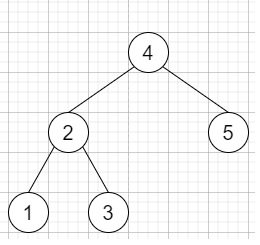# Find the closest element in Binary Search Tree in C++

C++Server Side ProgrammingProgramming

#### C in Depth: The Complete C Programming Guide for Beginners

45 Lectures 4.5 hours

#### Practical C++: Learn C++ Basics Step by Step

Most Popular

50 Lectures 4.5 hours

#### Master C and Embedded C Programming- Learn as you go

66 Lectures 5.5 hours

Suppose we have one binary search tree (BST) and another target value; we have to find k values in that given BST that are closest to the target. Here the target value is a floating-point number. We can assume k is always valid, and k ≤ total nodes.

So, if the input is liketarget = 3.714286, and k = 2, then the output will be [4,3]

To solve this, we will follow these steps −

• Define a function pushSmaller(), this will take node,stack st, and target,

• while node is not present, do −

• if val of node < target, then −

• insert node into st

• node := right of node

• Otherwise

• node := left of node

• Define a function pushLarger(), this will take node, stack st, target,

• while node is empty, do −

• if val of node >= target, then −

• insert node into st

• node := left of node

• Otherwise

• node := right of node

• From the main method do the following −

• Define an array ret

• Define one stack smaller

• Define one stack larger

• pushLarger(root, larger, target)

• pushSmaller(root, smaller, target)

• while k is non-zero, decrease k in each step, do −

• if smaller is not empty and (larger is empty or |target - value of top element of smaller| < |target - top element of larger|)

• curr = top element of smaller

• delete element from smaller

• insert val of curr at the end of ret

• pushSmaller(left of curr, smaller, target)

• Otherwise

• curr = top element of larger

• delete element from larger

• insert val of curr at the end of ret

• pushSmaller(right of curr, larger, target)

• return ret

## Example

Let us see the following implementation to get better understanding −

Live Demo

#include <bits/stdc++.h>
using namespace std;
void print_vector(vector<auto> v){
for(int i = 0; i<v.size(); i++){
cout << v[i] << ", ";
}
cout << "]"<<endl;
}
class TreeNode{
public:
int val;
TreeNode *left, *right;
TreeNode(int data){
val = data;
left = NULL;
right = NULL;
}
};
void insert(TreeNode **root, int val){
queue<TreeNode*> q;
q.push(*root);
while(q.size()){
TreeNode *temp = q.front();
q.pop();
if(!temp->left){
if(val != NULL)
temp->left = new TreeNode(val);
else
temp->left = new TreeNode(0);
return;
}
else{
q.push(temp->left);
}
if(!temp->right){
if(val != NULL)
temp->right = new TreeNode(val);
else
temp->right = new TreeNode(0);
return;
}
else{
q.push(temp->right);
}
}
}
TreeNode *make_tree(vector<int> v){
TreeNode *root = new TreeNode(v);
for(int i = 1; i<v.size(); i++){
insert(&root, v[i]);
}
return root;
}
class Solution {
public:
vector<int> closestKValues(TreeNode* root, double target, int k){
vector<int> ret;
stack<TreeNode*> smaller;
stack<TreeNode*> larger;
pushLarger(root, larger, target);
pushSmaller(root, smaller, target);
while (k--) {
if (!smaller.empty() && (larger.empty() || (abs(target - smaller.top()->val) < abs(target - larger.top()->val)))) {
TreeNode* curr = smaller.top();
smaller.pop();
ret.push_back(curr->val);
pushSmaller(curr->left, smaller, target);
}
else {
TreeNode* curr = larger.top();
larger.pop();
ret.push_back(curr->val);
pushLarger(curr->right, larger, target);
}
}
return ret;
}
void pushSmaller(TreeNode* node, stack <TreeNode*>& st, double target){
while (node) {
if (node->val < target) {
st.push(node);
node = node->right;
}
else {
node = node->left;
}
}
}
void pushLarger(TreeNode* node, stack <TreeNode*>& st, double target){
while (node) {
if (node->val >= target) {
st.push(node);
node = node->left;
}
else
node = node->right;
}
}
};
main(){
Solution ob;
vector<int> v = {4,2,5,1,3};
TreeNode *root = make_tree(v);
print_vector(ob.closestKValues(root, 3.7142, 2));
}

## Input

{4,2,5,1,3}, 3.7142, 2

## Output

[4, 3, ]